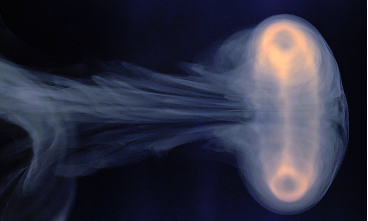# sandbox/Antoonvh/hoek.cA ring vortex. Image courtesy of Giesbert Nijhuis

# Hoek’s Vortex-ring generator

Rather than forcing a fluid though a circular opening, Bart Hoek proposed to force an opening though a fluid in order to generate a ring vortex. On this page we study this idea using the Navier-Stokes solver with the axisymmetric approximation.

#include "axi.h"
#include "navier-stokes/centered.h"
#include "fractions.h"
#include "view.h"
#include "particles.h"

The setup consist of a flat, circular plate (Radius Ro) with a circular opening (radius Ri) that moves for a time tc with a characteristic velocity U1.

#define U_V (2*U1*(t < tc)*sin(t*pi/tc))
#define PLATE (w - (fabs(x - xp)) - (y > Ro) - (y < Ri))
scalar plt[];

double tc = 1;   //Moving time
double w = 0.1;  //plate width
double U1 = -1;  //Plate velocity
double Ri = 1;   //Opening radius
double Ro = 5;   //The plate radius
double xp = -3;  //The plate's initial position

The domain is 30Ri  30Ri.

int main(){
L0 = 30;
X0 = -L0/2;
init_grid(1 << 7);
run();
}

The setup is seeded with tracer particles, they are placed close to the opening, within a disk or radius and depth 1.5\timesRi. Furthermore, we tell the adaptation algorithm that the plate is described by a volume fraction field.

event init(t = 0){
init_particles_2D_square_grid(60, xp - 1, 1.51, 1.5);
int j = 0;
while (j < n_part)
loc[j++].z = noise()*pi;
plt.prolongation = plt.refine = fraction_refine;
}

The plate moves and enforces a velocity via Stephane’s trick’.

event moving_plate(i++){
xp += dt*U_V;
fraction(plt, PLATE);
foreach(){
u.y[] *= (1-plt[]);
u.x[] = u.x[]*(1 - plt[]) + plt[]*U_V;
}
}

adapt_wavelet({plt, u.x, u.y}, (double[]){0.005, 0.05, 0.05}, 10);

## Output

We output a movie of the resulting flow. It displays:

• A slice of the moving plate as a light gray box (upper half).
• The vorticity field (upper half)
• The used grid (lower half)
• 3600 particles that trace the flow.

Remember that the flow is two-dimensional (u = u(z,r))

Two vortex ring emerge!

For the movie we need to use a modified version of the scatter() function to visualize the axisymmetric vortex ring.

// This is copied from draw.h
static void glvertex3d (bview * view, double x, double y, double z) {
if (view->map) {
coord p = {x, y, z};
view->map (&p);
glVertex3d (p.x, p.y, p.z);
}
else
glVertex3d (x, y, z);
}

struct _scatter {
coord * loc;    // Coordinates
float s, pc, coefs; // point size, colour and distance attenuation coefs.
};

void glPointParameterfv (GLenum pname, const GLfloat * params); //function protopyte

trace
void scatter3Din2D(struct _scatter p){// Modified from scatter.h
bview * view = draw();
if (!p.coefs){ // A guess:
p.coefs = 0.01;
p.coefs = 0.2;
p.coefs = 0.5;
}
glPointParameterfv(GL_POINT_DISTANCE_ATTENUATION, p.coefs);
if (p.pc)
glColor3f(p.pc, p.pc, p.pc);
if (!p.s)
p.s = 20;
glPointSize(p.s);
glBegin (GL_POINTS);
for (int j = 0; j < n_part; j++)
glvertex3d (view, p.loc[j].x, p.loc[j].y*cos(loc[j].z), p.loc[j].y*sin(loc[j].z));
glEnd();
view->ni++;
}

This is the movie-making event.

event mov (t += 0.0125){
scalar omega[];
vorticity (u, omega);
view (fov = 8, theta = -.75, tx = 0.05, width = 900, samples = 1);
double std = statsf (omega).stddev;
foreach(){
if (fabs(omega[])*2 < std || plt[] > 0.5)
omega[] = nodata; //Transparant
}
clear();
squares ("omega", map = cool_warm, min = -6*std, max = 6*std);
draw_vof ("plt", lw = 2);
draw_vof ("plt", filled = 1, fc = {0.7,0.7,0.7});
scatter3Din2D (loc, s = 6, pc = {0.2,0.2,0.2});
mirror ({0,-1})
cells();
save ("movie.mp4");
}

event stop (t = 6);`